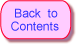# Puzzles A31 ... A40

### Part A — Loop Problems

These puzzles involve functions that contain loops.

#### Puzzle A31 — function to print a line of N stars

`[E-3]`Write a function that prints a line of N stars followed by an end of line. N is the parameter of the function. Here is the output of the function when N is 8:

```********
```

Here is a skeleton of the function and a `main()` program that exercises it:

```#include <stdio.h>

void starLine( int n )
{
}

int main(int argc, char *argv[])
{
starLine( 8 );

system("PAUSE");
return 0;
}
```

#### Puzzle A32 — Centered Row

`[E-5]`Write ```center(char ch, int count, int length)``` which prints `count` number of characters in the center of a line of `length` characters. Make the other characters of the line blank (or dot, for debugging purposes.) Here is the output for ```center('*', 5, 13)```

```....*****....
```

Here is a testing framework:

```#include <stdio.h>

void center(char ch, int count, int length)
{
}

int main(int argc, char *argv[])
{
center('*', 5, 13);

system("PAUSE");
return 0;
}
```

#### Puzzle A33 — print an R by C block of stars

`[E-3]`Write a function that prints a block of R rows of C stars. If R is 5 and C is 7 the function prints:

```*******
*******
*******
*******
*******
*******
*******
```

Write the function so that it uses `starLine()` from the first puzzle. Here is a skeleton of the function and a `main()` program that exercises it:

```#include <stdio.h>

void starLine( int n )
{
}

void starBlock( int rows, int cols )
{
}

int main(int argc, char *argv[])
{
starBlock( 5, 7 );

system("PAUSE");
return 0;
}
```

#### Puzzle A34 — Left Pyramid

`[E-5]`Write a function that prints a pyramid of B blocks. Each block is an N by 2N-1 rectangle, starting with N=1 and going up to N=B:

```*
***
***
*****
*****
*****
*******
*******
*******
*******
*********
*********
*********
*********
*********
***********
***********
***********
***********
***********
***********
```

Use the previous function `starBlock()`, to build the pyramid.

```#include <stdio.h>

void starLine( int n )
{
}

void starBlock( int rows, int cols )
{
}

void pyramid( int blocks )
{
}

int main(int argc, char *argv[])
{
pyramid( 7 );

system("PAUSE");
return 0;
}
```

#### Puzzle A35 — Centered Pyramid

`[E-7]`Modify the previous program so that it prints a centered pyramid:

```    *
***
***
*****
*****
*****
*******
*******
*******
*******
*********
*********
*********
*********
*********
```

Use the function `center()` from the second puzzle. You will need to modify `pyramid()` and `starBlock()`.

#### Puzzle A36 — print centered triangle

`[E-5]`Write a function that prints a centered triangle created by printed several centered rows of a specified character. The parameters to the function are the number of rows and the character to print. Use `center()` .

```    *
***
*****
*******
*********
```

Each row of the triangle has an odd number of characters.

```#include <stdio.h>

void center(char ch, int count, int length)
{
}

void triangle(char ch, int rows)
{
}

int main(int argc, char *argv[])
{
triangle('*', 5);

system("PAUSE");
return 0;
}
```

#### Puzzle A37 — print centered truncated triangle

`[E-5]`Write a function that prints a centered triangle with a base of N characters and a top row of T characters. The triangle is centered in a row of length number of characters (although trailing blanks are not printed.)

```      ***
*****
*******
*********
```

Each row of the triangle has an odd number of characters.

```#include <stdio.h>

void center(char ch, int count, int length)
{
}

void truncTriangle(char ch, int base, int top, int length)
{
}

int main(int argc, char *argv[])
{
truncTriangle('*', 9, 3, 17);

system("PAUSE");
return 0;
}
```

#### Puzzle A38 — print a shaggy centered truncated triangle

`[M-10]`As above, write a function that prints a centered triangle with a base of N characters and a top row of T characters. But now, use a different character for the first half of each line, the center, and the last half of each line.

```          ///|\\\
////|\\\\
/////|\\\\\
//////|\\\\\\
///////|\\\\\\\
////////|\\\\\\\\
```

Each row of the triangle has an odd number of characters. You will have to modify `center()`.

```#include <stdio.h>

void center(char left, char cent, char right, int count, int length)
{
}

void shaggyTruncTriangle(char left, char cent, char right, int base, int top, int length)
{
}

int main(int argc, char *argv[])
{
shaggyTruncTriangle('/', '|', '\\', 17, 7, 27);

system("PAUSE");
return 0;
}
```

The character constant `'\\' `in the above means just a single backslash. Since backslash is used as an escape character, two in a row are needed to ask for just one.

#### Puzzle A39 — Pine Tree

`[M-10]`Use `shaggyTruncTriangle()` in a function that prints a pine tree.

```        |
/|\
/|\
//|\\
///|\\\
//|\\
///|\\\
////|\\\\
///|\\\
////|\\\\
/////|\\\\\
////|\\\\
/////|\\\\\
//////|\\\\\\
/////|\\\\\
//////|\\\\\\
///////|\\\\\\\
//////|\\\\\\
///////|\\\\\\\
////////|\\\\\\\\
|||
|||
|||
```
```void center(char left, char cent, char right, int count, int length)
{
}

void shaggyTruncTriangle(char left, char cent, char right, int base, int top, int line)
{
}

void pineTree( int tiers )
{
}

int main(int argc, char *argv[])
{
pineTree(8);

system("PAUSE");
return 0;
}
```

#### Puzzle A40 — Solid Circle

`[H-10]`Write a function that prints a solid circle of stars.

```      *******
***********
*************
***************
***************
*****************
*****************
*****************
*****************
*****************
*****************
*****************
***************
***************
*************
***********
*******
```

Do this using a doubly nested loop that iterates through all rows and all columns. If the distance a particular row and column is from the center of the circle is less than the radius, print a star. Otherwise print a space. Use the Pythagorean theorem to calculate the distance squared. The center of the circle is at (row, col) == (radius, radius). Don't use `center()` for this.

```#include <stdio.h>— Return to the main contents page